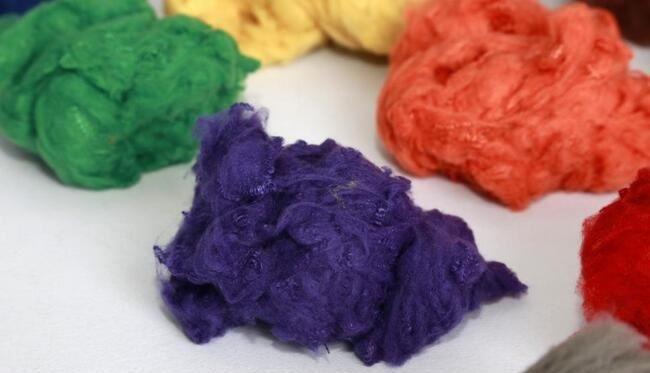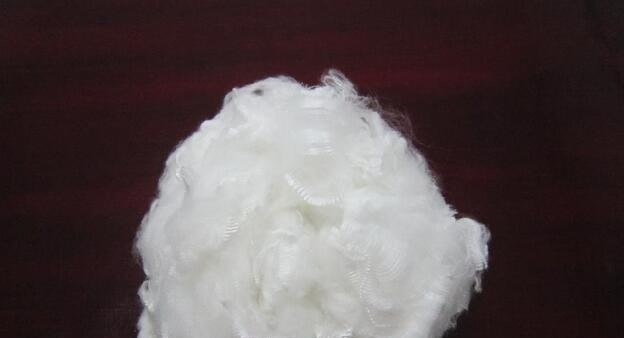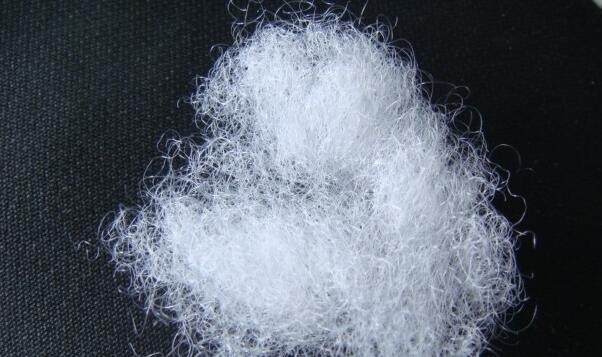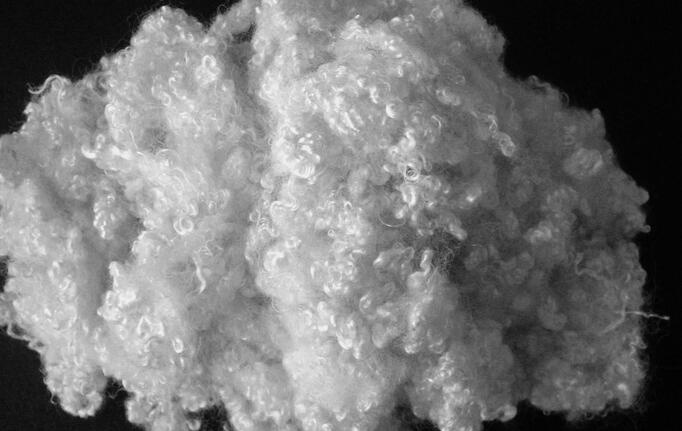|

# 2016涤纶短纤价格解析

涤纶短纤具有好的耐磨性和吸水性，用途广泛，在人们的生活中一直被广泛流传着，在市面上是一种很抢手的面料，那涤纶短纤价格贵不贵呢？接下来小编就给消费者朋友详细的介绍一下2016涤纶短纤价格情况。2016涤纶短纤价格解析

产品报价：供应1.4D－70D彩色涤纶短纤？7000.00一吨。

产品报价：阻燃涤纶短纤？14000.00一吨

涤纶短纤的特性

耐磨性：涤纶的耐磨性仅次于锦纶，在合成纤维中居第二位。

吸水性：涤纶的吸水回潮率低，绝缘性能好，但由于吸水性低，摩擦产生的静电大，染色性能较差。

涤纶短纤的用途

涤纶短纤产品主要用于棉纺行业，单独纺纱或与棉、粘胶纤维、麻、毛、维纶等混纺，所得纱线用于服装织布为主，还可用于家装面料，包装用布，充填料和保暖材料。涤纶短纤产家介绍

宁波大发化纤有限公司

宁波大发化纤有限公司创建于1995年，地处杭州湾跨海大桥南岸的杭州湾新区，注册资金1200万美元，总资产10亿元。公司占地面积335亩，员工1000余人，工程技术人员175人，固定资产6亿元，拥有国内先进的再生中空涤纶短纤维生产线9条，年产能26万吨，是全球最大产能的再生中空涤纶短纤维生产企业之一。

公司是利用回收的PET饮料瓶及其他PET类废料、聚酯泡料、化纤废丝、块等为原料加工生产再生中空涤纶短纤维的绿色环保企业。公司有一支专业的技术研发团队，近几年来，自主研发出一套完整的PET饮用瓶分拣、粉碎、清洗生产线和废PET原料加工的专用技术设备，能将PET饮用瓶纺制成优质差别化再生涤纶短纤维。2008年完成了主持制定的《再生涤纶短纤维》行业标准。在中国乃至全球“再生中空短纤”行业中，其研发能力、生产能力、行业知名度、品种、效益等主要指标均居于领先地位。2012年又制定了《再生阻燃涤纶短纤维》的行业标准。公司开发的8孔中空再生涤纶短纤维等3个项目列入国家重点新产品计划；再生中空脱油硬质涤纶纤维等4个项目列入国家火炬计划项目。公司每年申报大量专利，到目前为止，公司已经申报专利222项，获得批准192项，其中发明专利21项。2007年被认定为“浙江省专利示范企业”，2008年公司还被认定为“国家高新技术企业”。

多年来，坚定不移地走科技创新之路，同时坚持 “大发化纤大家发”这一企业文化，其中一发“合作伙伴发”就是要保证客户、银行和消费者的利益。公司把创造财富、保护环境、关注社会作为自己的崇高责任。对外遵纪守法，诚实守信，严守“质量第一、客户至上”的经营理念；对内以人为本，注重科技创新，受到广大客户和社会各界的一致认同。公司生产的“大发牌”再生中空涤纶短纤维被评浙江省名牌产品、浙江省出口名牌、“大发”商标认定为中国驰名商标，“大发化纤”获得浙江省知名商号。安徽碧深高纤有限公司

安徽碧深高纤有限公司成立于2011年，属民营企业。地处安徽宣城市郎溪县毕桥镇，地理位置优越，交通十分便利。工厂占地面积50000平方米，现有建筑面积36000多平方米，员工200多人，固定资产1.35个亿。主要生产各种规格、各种颜色的涤纶短纤维，目前拥有两条生产流水线，预备2015年再上两条生产线，目前年产涤纶短纤维30000吨，专供各大、中、棉纺毛纺公司。公司设备精良，工艺先进，化验检测手段齐全，技术力量雄厚，具有大批量、多系列、多品种、高质量的生产规模和高水平的管理能力。公司的经营方针建立在全心全意为客户服务和对产品质量永不止息的追求上，以其灵活、周到的服务满足客户的要求。

公司自成立以来，本着“团结、真诚、务实、敬业“的企业精神，坚持走“科技兴企“之路，向产品要质量，向管理要效益，充分调动和不断激励公司全体员工，以稳健高效为目的，逐步推进企业蓬勃发展，使企业逐步走上规范化、高效化发展道路。

今后，公司还将扩大规模，增强高科技投入，调整企业产品结构，优化资源管理、不断追求卓越品质，提高公司在市场上 的竞争优势。公司二期项目，总设备投资6000万元，将建成4条生产流水线，年产涤纶短纤维60000吨。公司三期项目，预计总投资3.2个亿，将建成化纤原料，涤纶纤维，涤纶纱线全面结合发展道路 安徽碧深高纤有限公司是涤纶纤维、涤纶短纤、化纤造粒、PET、废旧纺织品等产品专业生产加工的公司，拥有完整、科学的质量管理体系。安徽碧深高纤有限公司的诚信、实力和产品质量获得业界的认可。欢迎各界朋友莅临参观、指导和业务洽谈。江阴协达特种化纤有限公司

江阴协达特种化纤有限公司成立于2006年3月，公司座落于位于经济发达、交通便利的江苏省江阴市长泾镇工业集中区，东临通锡公路S19，北靠 江阴华西村，北距张家港18公里，南距无锡20公里，近靠沿江高速S38和云顾线，距离上海仅160公里。公司有3条涤纶短纤生产线，日产量为110吨 ，年产量达30000多吨。

公司主营高压纺有色涤纶短纤维的生产和销售，产品规格从1.4D－15D；纤维长度32MM－150MM。常规颜色主要有：普黑中化、中纤黑中化、特 黑中化，其他颜色都是定喷，颜色有：大红、藏青、宝蓝、松子灰、铁灰、天蓝、天空蓝、粉红、玫红、橘红、咖啡、紫罗兰，同时公司可以根 据客户的要求生产各种有色纤维， 公司产品主要应用于纺纱，热轧布，喷胶棉，无纺布，汽车内饰，地毯，床上用品，填充料，喷胶棉等领域 。

公司集研发、生产、销售、外贸进出口于一体的技术型企业， 公司以＂质量就是生命＂为经营理念，狠抓产品质量， 以先进的企业文化，严格 的管理制度，科学的考核办法，确保企业能够长远发展，不断进取。

公司拥有进出口经营权，经营稳健、开发实力雄厚、质量稳定、深受国内外客商青睐，已经成功进入东南亚，美国，欧洲，南美，中东等 国家和地区。

公司先后被评为江阴市明星企业、无锡市明星企业、无锡市重合同守信用、江苏省AAA级信用企业、江苏省明星企业。

`声明：本文由入驻焦点开放平台的作者撰写，除焦点官方账号外，观点仅代表作者本人，不代表焦点立场错误信息举报电话： 400-099-0099，邮箱：jubao@vip.sohu.com，或点此进行意见反馈，或点此进行举报投诉。`A B C D E F G H J K L M N P Q R S T W X Y Z
A - B - C - D - E
• A
• 鞍山
• 安庆
• 安阳
• 安顺
• 安康
• 澳门
• B
• 北京
• 保定
• 包头
• 巴彦淖尔
• 本溪
• 蚌埠
• 亳州
• 滨州
• 北海
• 百色
• 巴中
• 毕节
• 保山
• 宝鸡
• 白银
• 巴州
• C
• 承德
• 沧州
• 长治
• 赤峰
• 朝阳
• 长春
• 常州
• 滁州
• 池州
• 长沙
• 常德
• 郴州
• 潮州
• 崇左
• 重庆
• 成都
• 楚雄
• 昌都
• 慈溪
• 常熟
• D
• 大同
• 大连
• 丹东
• 大庆
• 东营
• 德州
• 东莞
• 德阳
• 达州
• 大理
• 德宏
• 定西
• 儋州
• 东平
• E
• 鄂尔多斯
• 鄂州
• 恩施
F - G - H - I - J
• F
• 抚顺
• 阜新
• 阜阳
• 福州
• 抚州
• 佛山
• 防城港
• G
• 赣州
• 广州
• 桂林
• 贵港
• 广元
• 广安
• 贵阳
• 固原
• H
• 邯郸
• 衡水
• 呼和浩特
• 呼伦贝尔
• 葫芦岛
• 哈尔滨
• 黑河
• 淮安
• 杭州
• 湖州
• 合肥
• 淮南
• 淮北
• 黄山
• 菏泽
• 鹤壁
• 黄石
• 黄冈
• 衡阳
• 怀化
• 惠州
• 河源
• 贺州
• 河池
• 海口
• 红河
• 汉中
• 海东
• I
• J
• 晋中
• 锦州
• 吉林
• 鸡西
• 佳木斯
• 嘉兴
• 金华
• 景德镇
• 九江
• 吉安
• 济南
• 济宁
• 焦作
• 荆门
• 荆州
• 江门
• 揭阳
• 金昌
• 酒泉
• 嘉峪关
K - L - M - N - P
• K
• 开封
• 昆明
• 昆山
• L
• 廊坊
• 临汾
• 辽阳
• 连云港
• 丽水
• 六安
• 龙岩
• 莱芜
• 临沂
• 聊城
• 洛阳
• 漯河
• 娄底
• 柳州
• 来宾
• 泸州
• 乐山
• 六盘水
• 丽江
• 临沧
• 拉萨
• 林芝
• 兰州
• 陇南
• M
• 牡丹江
• 马鞍山
• 茂名
• 梅州
• 绵阳
• 眉山
• N
• 南京
• 南通
• 宁波
• 南平
• 宁德
• 南昌
• 南阳
• 南宁
• 内江
• 南充
• P
• 盘锦
• 莆田
• 平顶山
• 濮阳
• 攀枝花
• 普洱
• 平凉
Q - R - S - T - W
• Q
• 秦皇岛
• 齐齐哈尔
• 衢州
• 泉州
• 青岛
• 清远
• 钦州
• 黔南
• 曲靖
• 庆阳
• R
• 日照
• 日喀则
• S
• 石家庄
• 沈阳
• 双鸭山
• 绥化
• 上海
• 苏州
• 宿迁
• 绍兴
• 宿州
• 三明
• 上饶
• 三门峡
• 商丘
• 十堰
• 随州
• 邵阳
• 韶关
• 深圳
• 汕头
• 汕尾
• 三亚
• 三沙
• 遂宁
• 山南
• 商洛
• 石嘴山
• T
• 天津
• 唐山
• 太原
• 通辽
• 铁岭
• 泰州
• 台州
• 铜陵
• 泰安
• 铜仁
• 铜川
• 天水
• 天门
• W
• 乌海
• 乌兰察布
• 无锡
• 温州
• 芜湖
• 潍坊
• 威海
• 武汉
• 梧州
• 渭南
• 武威
• 吴忠
• 乌鲁木齐
X - Y - Z
• X
• 邢台
• 徐州
• 宣城
• 厦门
• 新乡
• 许昌
• 信阳
• 襄阳
• 孝感
• 咸宁
• 湘潭
• 湘西
• 西双版纳
• 西安
• 咸阳
• 西宁
• 仙桃
• 西昌
• Y
• 运城
• 营口
• 盐城
• 扬州
• 鹰潭
• 宜春
• 烟台
• 宜昌
• 岳阳
• 益阳
• 永州
• 阳江
• 云浮
• 玉林
• 宜宾
• 雅安
• 玉溪
• 延安
• 榆林
• 银川
• Z
• 张家口
• 镇江
• 舟山
• 漳州
• 淄博
• 枣庄
• 郑州
• 周口
• 驻马店
• 株洲
• 张家界
• 珠海
• 湛江
• 肇庆
• 中山
• 自贡
• 资阳
• 遵义
• 昭通
• 张掖
• 中卫

1室1厅1厨1卫1阳台

1
2
3
4
5

0
1
2

1

1

0
1
2
3报名成功，资料已提交审核A B C D E F G H J K L M N P Q R S T W X Y Z
A - B - C - D - E
• A
• 鞍山
• 安庆
• 安阳
• 安顺
• 安康
• 澳门
• B
• 北京
• 保定
• 包头
• 巴彦淖尔
• 本溪
• 蚌埠
• 亳州
• 滨州
• 北海
• 百色
• 巴中
• 毕节
• 保山
• 宝鸡
• 白银
• 巴州
• C
• 承德
• 沧州
• 长治
• 赤峰
• 朝阳
• 长春
• 常州
• 滁州
• 池州
• 长沙
• 常德
• 郴州
• 潮州
• 崇左
• 重庆
• 成都
• 楚雄
• 昌都
• 慈溪
• 常熟
• D
• 大同
• 大连
• 丹东
• 大庆
• 东营
• 德州
• 东莞
• 德阳
• 达州
• 大理
• 德宏
• 定西
• 儋州
• 东平
• E
• 鄂尔多斯
• 鄂州
• 恩施
F - G - H - I - J
• F
• 抚顺
• 阜新
• 阜阳
• 福州
• 抚州
• 佛山
• 防城港
• G
• 赣州
• 广州
• 桂林
• 贵港
• 广元
• 广安
• 贵阳
• 固原
• H
• 邯郸
• 衡水
• 呼和浩特
• 呼伦贝尔
• 葫芦岛
• 哈尔滨
• 黑河
• 淮安
• 杭州
• 湖州
• 合肥
• 淮南
• 淮北
• 黄山
• 菏泽
• 鹤壁
• 黄石
• 黄冈
• 衡阳
• 怀化
• 惠州
• 河源
• 贺州
• 河池
• 海口
• 红河
• 汉中
• 海东
• I
• J
• 晋中
• 锦州
• 吉林
• 鸡西
• 佳木斯
• 嘉兴
• 金华
• 景德镇
• 九江
• 吉安
• 济南
• 济宁
• 焦作
• 荆门
• 荆州
• 江门
• 揭阳
• 金昌
• 酒泉
• 嘉峪关
K - L - M - N - P
• K
• 开封
• 昆明
• 昆山
• L
• 廊坊
• 临汾
• 辽阳
• 连云港
• 丽水
• 六安
• 龙岩
• 莱芜
• 临沂
• 聊城
• 洛阳
• 漯河
• 娄底
• 柳州
• 来宾
• 泸州
• 乐山
• 六盘水
• 丽江
• 临沧
• 拉萨
• 林芝
• 兰州
• 陇南
• M
• 牡丹江
• 马鞍山
• 茂名
• 梅州
• 绵阳
• 眉山
• N
• 南京
• 南通
• 宁波
• 南平
• 宁德
• 南昌
• 南阳
• 南宁
• 内江
• 南充
• P
• 盘锦
• 莆田
• 平顶山
• 濮阳
• 攀枝花
• 普洱
• 平凉
Q - R - S - T - W
• Q
• 秦皇岛
• 齐齐哈尔
• 衢州
• 泉州
• 青岛
• 清远
• 钦州
• 黔南
• 曲靖
• 庆阳
• R
• 日照
• 日喀则
• S
• 石家庄
• 沈阳
• 双鸭山
• 绥化
• 上海
• 苏州
• 宿迁
• 绍兴
• 宿州
• 三明
• 上饶
• 三门峡
• 商丘
• 十堰
• 随州
• 邵阳
• 韶关
• 深圳
• 汕头
• 汕尾
• 三亚
• 三沙
• 遂宁
• 山南
• 商洛
• 石嘴山
• T
• 天津
• 唐山
• 太原
• 通辽
• 铁岭
• 泰州
• 台州
• 铜陵
• 泰安
• 铜仁
• 铜川
• 天水
• 天门
• W
• 乌海
• 乌兰察布
• 无锡
• 温州
• 芜湖
• 潍坊
• 威海
• 武汉
• 梧州
• 渭南
• 武威
• 吴忠
• 乌鲁木齐
X - Y - Z
• X
• 邢台
• 徐州
• 宣城
• 厦门
• 新乡
• 许昌
• 信阳
• 襄阳
• 孝感
• 咸宁
• 湘潭
• 湘西
• 西双版纳
• 西安
• 咸阳
• 西宁
• 仙桃
• 西昌
• Y
• 运城
• 营口
• 盐城
• 扬州
• 鹰潭
• 宜春
• 烟台
• 宜昌
• 岳阳
• 益阳
• 永州
• 阳江
• 云浮
• 玉林
• 宜宾
• 雅安
• 玉溪
• 延安
• 榆林
• 银川
• Z
• 张家口
• 镇江
• 舟山
• 漳州
• 淄博
• 枣庄
• 郑州
• 周口
• 驻马店
• 株洲
• 张家界
• 珠海
• 湛江
• 肇庆
• 中山
• 自贡
• 资阳
• 遵义
• 昭通
• 张掖
• 中卫• 手机• 分享
• 设计
免费设计
• 计算器
装修计算器
• 入驻
合作入驻
• 联系
联系我们
• 置顶
返回顶部